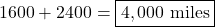## Give brainiest if right!! Two planes travel in opposite directions from the same airport. The first plane travels at a spe

Question

Give brainiest if right!!

Two planes travel in opposite directions from the same airport. The
first plane travels at a speed of 320 mph, and the second plane’s
speed is 1.5 times greater. What is the distance between the two
planes after 5 hours?

in progress 0
5 months 2021-08-22T14:23:52+00:00 2 Answers 3 views 0

## Answers ( )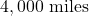Step-by-step explanation:

Let’s say that the two planes travel at point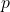. Without loss of generality, let’s say that the first plane travels left and the second plane travels right.

Distance is given by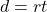, where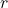is rate or speed andis time.

If the first plane travels at a speed of 320 mph and the second plane travels 1.5 times this, the second plane must travel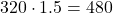mph.

Using, the first plane must travel: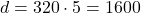miles

The second plane must travel: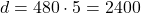miles

However, these are the planes’ respective distances from point. Since they travelled in opposite directions, the total distance between them is equal to